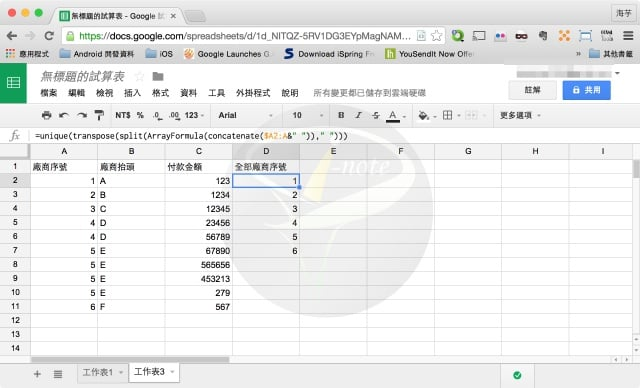◎◎ 網站 小檔案◎◎

■ 網站性質：檔案共享、雲端硬碟、線上文書`=unique(transpose(split(ArrayFormula(concatenate(\$A2:A&" "))," ")))``=unique(transpose(split(ArrayFormula(concatenate(\$B2:B&" "))," ")))`

`=SUMIF(A2:A11,D2,C2:C11)``=SUMIF(A2:A11,D3,C2:C11)`

Subscribe
Notify of